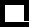The Krib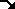Plumbing and Filtration [E-mail]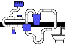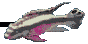## Total equivalent head of plumbing

### by swhite-at-bach.udel.edu (Stephen H White) Date: Wed, 31 Mar 1993 Newsgroup: rec.aquaria

```In article <27915-at-hacgate.SCG.HAC.COM> larisa-at-tcville.edsg.hac.com writes:
>Could someone please remind me of the formula which computes total
>equivalent vertical head based on measurements of vertical and horizontal
>runs?  I seem to recall something like this: "a horizontal run takes as
>much work to pump through as a vertical run of 0.4 times its length."
>
>I need to find the equivalent vertical head of my overall plumbing.  Also,
>if you remember how to compute pressure at arbitrary head based on a
>pressure measurement at a given head, feel free to include the info.
>
>Follow-ups to rec.aquaria unless you select otherwise.
>
>-- Larisa
>larisa-at-tcville.edsg.hac.com
>

These formulas may help:

head is in units of feet
pressure is in psi

Bernoulli's Equation can be used to determine the total head of a system:

Bernoulli's Equation:
pres(1)/spwt + vel(1)^2/2GR + EL(1) + WI ==== pres(2)/spwt + vel(2)^2/2GR +
EL(2) + WO + TFHL
where:
pres(1) = pressure at point 1 [Pascals]
pres(2) = pressure at point 2 [Pascals]
spwt = specific weight of fluid, typically water [Fresh H2O=9782 N/m^3 -at-20degC]
vel(1) = fluid velocity at point 1 [m/s]
vel(2) = fluid velocity at point 2 [m/s]
EL(1) = Elevation at point 1 relative to a chosen datum(EL=0),  [m]
EL(2) = Elevation at point 2 [m]
WI = work in; solving for this will produce total head required by a pump
WO = work out, usually ignored unless there are devices in system which
are storing energy, ie accumulators, etc...
GR = 9.806 m/s^2, the acceleration of gravity
TFHL = the total fluid head loss due to frictionary forces [m]

FHL(I) = FF*TEPL*VEL^2/(2GR*DIA)
where:
FF = friction factor for ith section of pipe
TEPL = total equivalent pipe length for ith pipe section; this includes the
total straight pipe length plus equivalent pipe lengths from pipe
components such as elbows, reducers, etc... [m]
VEL = velocity through ith pipe section [m/s]
DIA = diameter pf ith pipe section (id)   [m]

TFHL = FHL(1)+FHL(2)+...+FHL(n-1)+FHL(n)   ---> total fluid head loss

Friction factors are determined through the use of a moody's diagram.
In order to determine the friction factor from the graph, the reynold's #
must be calculated, as well as the relative roughness of the pipe.
RE=VEL(I)*DIA(I)/KIVIS
where:
KIVIS=kinematic viscosity of fluid, use 1.3E-6 N/m^2 for freshwater -at-20C.
if RE<2000 then FF=64/RE
else use a moody diagram.

Most people won't have a moody diagram handy;)
therefore, if RE>2000 use a FF of 0.04 for calculations.  This is a conservativevalue for FF for most applications.
RELATIVE ROUGHNESS, RR=0.00006/DIA(I) for steel pipe.
For copper pipe use the smooth pipe line on the moody diagram.

Volumetric Flow=AREA(I)*VEL(I)

All of this is very simple....NOT!   ;)

Friction plays a very key role in plumbing applications. Once the total head
is calculated, (WI=Total Head, from bernoulli's EQN) the pump can be selected.
This is also a very complicated process, but for the sake of brevity, choose
a pump which will is rated for a head close to the calculated total head. Don't
oversize the pump too much, as this will result in a less efficent pump setup.

#############################################################
#					                    #
#       o___            Stephen H. White                    #
#     _ *> /  _         Internet (swhite-at-brahms.udel.edu)   #
#    (_)   \ (_)        University of Delaware              #
#							    #
#############################################################

```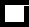Up to Plumbing and Filtration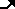The Krib This page was last updated 29 October 1998